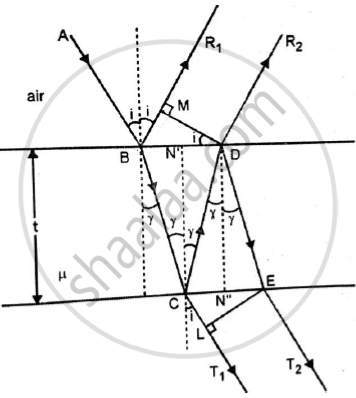# Derive the conditions of maxima and minima due to interference of light transmitted from thin film of uniform thickness. - Applied Physics 2

Derive the conditions of maxima and minima due to interference of light transmitted from thin film of uniform thickness.

#### SolutionConsider a thin film of uniform thickness (t) and R.I (μ)

On Reflected side,

The ray of light R1 and R2 will interfere.

The path difference between R1 and R2 is,
Δ = μ(BC + CD) − BG
BC = CD = t/cosr ..........(1)

Now, BD = (2t) tan r .......(2)

BM = BD sin i

BM = (2t) tan r sin i

BM = 2tμsinr(sinr / cosr)

BM = 2μt((sin^2r)/cosr) ..........(3)

Substituting (i) and (iii) in Δ :
Δ = μ(t / cosr + t / cosr)−2μt((sin2r) / cosr)
= 2μtcosr(1−sin^2r)
Δ = 2μtcosr
For transmitted system :
The transmitted rays CT1 and ET2 are also derived from the same incident ray AB and hence they are coherent.

Path difference = △ = μ(CD + DE) – CL
For constructive interference :

2μtcos r = n𝜆

For destructive interference :
2μtcos r = (2n – 1)λ/2

Concept: Interference in Thin Film of Constant Thickness Due to Reflected and Transmitted Light
Is there an error in this question or solution?
2016-2017 (June) CBCGS

Share